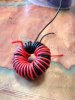# Impedance matching transformer

Discussion in 'Antennas, Feedlines, Towers & Rotors' started by W6OGC, Apr 3, 2015.1. Calculate it: calculate the inductance needed from L=X/(2.Pi.F); then calculate the number of turns needed for that inductance from the Al figure for that core.

I've never heard of a T-86-2 core so can't help you there.

For a T-106-2, the secondary would need 21 turns to produce a reactance of >500 Ohms at 14MHz; the primary would then need to be 15 turns.

Steve G3TXQ

2. ### W5DXPHam MemberQRZ Page

Sorry, I posted the following before I saw Steve's above posting.

We can use a decent antenna analyzer like the AIM4170D. Or we can use the published AL (uH/100T) values and a formula. The AL value for a T-80-2 is 55 uH/100T and the AL value for a T-94-2 is 84 uH/100T. The formula is:

Turns = 100*SQRT(L/AL) where the desired inductance L = XL/(2*pi*freq) and XL=5*Zload

3. Thanks, men. Splendid. What results if one doesn't have 5 times the load impedance?

It looks to me, poking around the Internet that, for power levels of 100 watts and less, no. 22 wire ought to be adequate on these cores.

4. Using the toroid calculator at http://toroids.info/T68-2.php you can enter a number of turns to find the corresponding inductance of the primary and secondary windings. Then, convert that inductance to a reactance using the usual formula. Since your load impedance is 100 ohms, you would want a reactance of at least 500 ohms. Only you know what your design frequency is and thus how many turns you will need on the core.

-Neil N0FN

5. This is starting to get exciting now. BTW, Steve, I fat fingered the core. I was thinking of the T-80-2.

I've been fiddling with your formulas and found at Kits and Parts, the details on all kinds of toroids, and a turns calculator that agreed right to the penny with my working your formula by hand! So easy, even a lawyer can do it.... once you show him how.

It looks to me like the T130-6 would be a better choice, as it is larger, will take the ~34 turns/24 turns required and is rated for 3-40 Mhz, better for my purposes for 7, 10 and possibly 14 Mhz. Any suggestions for something better?

6. I procured a larger toroid, T-130-2, as the smaller one wouldn't take enough turns using No. 22 wire.

For a frequency of 7.03 MHz, I figured 32 turns and 27 turns. The secondary has to be wound over the primary.To try this out, I connected a 100 ohm resistor across one winding and a SO-239 connector across the other. Using an antenna analyzer, it does not show the slightest impedance transformation, or anything. I expected it to show a 1:1 or near SWR at or very close to 7.03 Mhz.

Any idea where I have gone wrong?

7. ### KL7AJHam MemberQRZ Page

Yes that would work. You have to be careful though, because "conventionally" wound transformers at H.F. can have sharp resonances, which is why the broadbanded "transmission line transformer" was developed. There's a lot of good information out there on winding r.f. transformers in the ARRL Handbook and elsewhere.

eric

8. A big reason for using a transmission line transformer is to avoid coupling the input to the output by flux linkage thru the core. If the TLT has enough common mode impedance little flux is developed in the core minimizing power loss in the transformer. I thought it was a simple as that. 73, Pete

9. I don't understand what that means! What figures did you actually measure?

A 32:27 turns ratio is 1.18:1 and an impedance transformation ratio of 1.4:1. I thought you were trying to achieve 2:1?

Steve G3TXQ

Last edited: May 10, 2015
10. Sorry, I mistyped. I used 32 turns per the formula, and kitsandparts.com' helpful calculator, then divided that by 1.414 to get 21.7, or 22 turns, for frequency 7.03 and using a T130-2.

When I connected this to an analyzer, it showed very high Z, mostly over 350 ohms from 1-60 MHz, the range of the device, and SWR values to match, off the scale. Whatever I was expecting, it wasn't this.

Does the device shown in the photo resemble what it ought to look like?

Last edited: May 10, 2015